# 1st PUC Physics Previous Year Question Paper March 2019 (South)

Students can Download 1st PUC Physics Previous Year Question Paper March 2019 (South), Karnataka 1st PUC Physics Model Question Papers with Answers helps you to revise the complete Karnataka State Board Syllabus and score more marks in your examinations.

## Karnataka 1st PUC Physics Previous Year Question Paper March 2019 (South)

Time: 3.15 Hours
Max Marks: 70

General Instructions:

1. All parts are compulsory.
2. Draw relevant figure / diagram wherever necessary.
3. Numerical problems should be solved with relevant formulae.

Part – A

I. Answer ALL the following questions. ( 10 × 1 = 10 )

Question 1.
Write the dimensional formula for force.
[Force] = [M1 L1 T-2]

Question 2.
Write the SI unit of power.
SI unit of power is ‘watt’.

Question 3.
What is projectile motion?
A particle thrown into space at an angle and whose motion guided by the action of Earth’s gravity is called projectile motion.

Question 4.
Write relation between angular velocity and linear velocity.
v = Rω; where, v = Linear velocity and ω = angular velocity.Question 5.
Write expression for acceleration due to gravity at a height h from the surface of earth.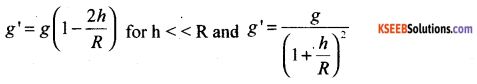where h – altitude, R – radius of the earth, g – acceleration due to gravity on the surface of the earth, g’ – acceleration due to gravity at an altitude.

Question 6.
Define stress.
Stress is the restoring force developed per unit area in a body when a deforming force is applied to it
Stress = restoring force / area.

Question 7.
Define angle of contact.
The angle between the normal reaction force and the contact force is known as the angle of contact.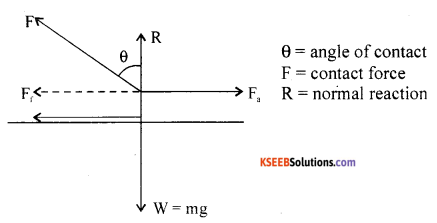where Ff – force of friction, Fa – applied force, W – weight of the body, R – normal reaction force, FR – resultant of force between force of friction and the reaction force.

Question 8.
Write ideal gas equation for one mole of gas.
PV = RT.Question 9.
State zeroth law of thermodynamics.
If two thermodynamic systems are in thermal equilibrium with third individually, then the systems are said to be in mutual thermal equilibrium with each other.
If TA = TC, TB = TC then TA = TB. where ‘T’ represents the temperatures and A, B, C represent the thermodynamic systems.

Question 10.
State law of equipartition of energy.
For gases in thermal equilibrium, the energy supplied to the gas is equally distributed among the degrees of freedom and molecules, so that energy per molecule per degree of freedom is
$$\left(\frac{1}{2}\right)$$ kBT where kB is Boltzmann’s constant.

part – B

II. Answer any five of the following questions : ( 5 × 2 = 10 )

Question 11.
Name any two fundamental forces in nature.

1. Gravitational force
2. Electro – weak force
3. Strong nuclear force

Question 12.
Write any two applications of dimensional analysis.
Dimensional analysis is used

1. To check the correctness of an equation
2. To express the unit of physical quantity from one system of unit into another.
3. To derive an equation.Question 13.
A body gets displacement of 5m in 2s, what is the average velocity?
Average velocity = $$\frac{\text { displacement }}{\text { time taken }}$$
i.e., v = $$\frac{5}{2}$$ = 2.5 ms-1

Question 14.
Define scalar product of two vectors.
Scalar product $$\overrightarrow{\mathrm{A}} \cdot \overrightarrow{\mathrm{B}}$$ = AB cosθ
where A = $$|\vec{A}|$$ and B = $$|\vec{B}|$$.

Question 15.
What is kinetic friction? Write the expression.
The ratio of kinetic friction to the normal reaction due to interacting surfaces is called coefficient of kinetic friction.
μk = $$\frac{F_{k}}{R}$$
Note: μk < μs

Question 16.
Define specific heat capacity of a substance.
The amount of heat required to raise the given mass of the substance through one kelvin of temperature is known as its thermal capacity.
i.e, thermal capacity of a substance = ms = $$\left(\frac{\mathrm{Q}}{\Delta \mathrm{T}}\right)$$
where ∆T = 1K.

Question 17.
Write any two differences between isothermal process and adiabatic process.
Isothermal process:

• In this process changes in pressure and volume of gas take place at a constant temperature.
• Boyle’s law holds good.
• PV = constant
• Generally this process is slow
• Change in the internal energy dU = 0
• Specific heat of gas in this process is infinity.
• Work done in this process is expressed in terms of changes in volume and constant temperature.
W = 2.303 μRT log $$\left(\frac{v_{2}}{v_{1}}\right)$$

• In this process, changes in pressure and volume take place under a thermal isolation.
• Boyle’s law does not hold good.
• PVγ = constant & γ = $$\frac{C_{p}}{C_{V}}$$
• Generally this process is fast.
• Change in entropy dS = 0 Internal energy of gas decreases on expansion and increases on compression.
• Specific heat of gas in this process remains zero.
• Work done in this process is expressed in terms of changes in temperature.
W = $$\frac{\mu R}{(\gamma-1)}$$ (T1 = T2)
i.e., Work done is measured in terms of fall in temperature or rise in temperature.Question 18.
Define frequency and period of oscillation.
The smallest interval of time after which the motion of the particle is repeated is called the period of oscillation. The time taken for one complete to and fro motion of a particle is known as the period of oscillation.
The number of times a particle executes a complete to and fro motion in one second is known as its frequency.

Part – C

III. Answer any Five of the following questions. ( 5 × 3 = 15 )

Question 19.
A body is moving in uniform circular motion. Derive an expression for centripetal acceleration.
Let $$\vec{r}$$ and $$\vec{r}^{\prime}$$ be the position vectors and $$\vec{v}$$ and $$\vec{v}^{\prime}$$ veIocities of the object when it is at point P and P’. By definition, velocity at a point is along the tangent at that point in the direction of the motion. Since the path is circular $$\vec{v}$$ is perpendicular to $$\vec{r}$$ and $$\vec{v}^{\prime}$$ is perpendicular to $$\vec{r}^{\prime}$$.
Therefore, ∆$$\vec{v}$$is perpendicular to ∆$$\vec{r}$$ Average acceleration $$\frac{\Delta \vec{v}}{\Delta t}$$ is perpendicular to ∆$$\vec{r}$$.
The magnitude of $$\vec{a}$$ is, by definition, given by $$|\vec{a}|=\lim _{\Delta t \rightarrow 0} \frac{|\Delta \vec{v}|}{\Delta t}$$
The triangle formed by the position vectors is similary to the triangle formed by the velocity vectors.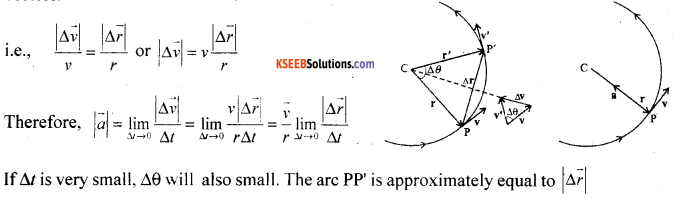lf ∆t is very small. ∆ will also small. The arc PP is approximately equal to $$|\Delta \vec{r}|$$
i.e., $$\lim _{\Delta t \rightarrow 0} \frac{|\Delta \vec{r}|}{\Delta t}$$ = v. Thus, centripetal acceleration $$|\vec{a}|=\frac{v}{r} v=\frac{v^{2}}{r}$$ and $$\vec{a}=\frac{v}{r} \frac{d \vec{r}}{d t}$$.
The centripetal acceleration is always directed towards the centre. The centripetal force = ma.Question 20.
State Newton’s second law of motion. Hence derive F= ma.
Newton’s II law of motion:
Statement: “The rate of change in the linear momentum of a body is directly proportional to the impressed force and takes place in the direction of force applied.
To show that $$\vec{F}=m \vec{a}$$.
Let m be the mass of the body. Let be the initial linear momentum. Let be the final linear momentum as a result of the impressed force.
By definition $$\lim _{\Delta t \rightarrow 0} \frac{\Delta \vec{p}}{\Delta t}=\frac{d \vec{p}}{d t}$$ where $$\Delta \vec{p}=\vec{p}_{f}-\vec{p}_{i}$$, and $$\frac{d \vec{p}}{d t}$$ is instantaneous acceleration.
From Newton’s II law of motion,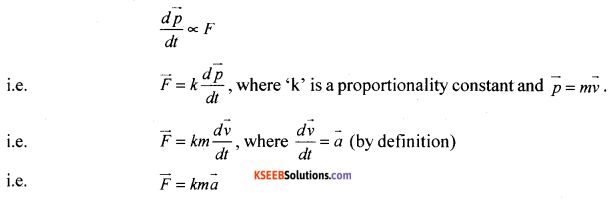But the magnitude of force $$|\overrightarrow{\mathrm{F}}|$$ is so defined that k = 1 : We denote $$|\vec{F}|=m|\vec{a}|$$
Thus F=ma and $$\vec{F}=m \vec{a}$$.

Question 21.
State work energy theorem with proof.
Let ‘K’ represent kinetic energy, we know that K = 1/2 mv2. the time rate of change of kinetic energy is given by =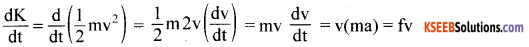i.e., $$\frac{\mathrm{d} \mathrm{K}}{\mathrm{dt}}=\mathrm{F} \frac{\mathrm{d} \mathrm{x}}{\mathrm{dt}}$$ where v = $$\frac{d x}{d t}$$ = time rate of displacement of particle.
or dK = Fdx
Integrating from the initial to final position,
we write $$\int_{k_{i}}^{k_{f}} \mathrm{d} \mathrm{K}=\int_{x_{i}}^{x_{f}} \mathrm{Fdx}$$
i.e., ( Kf – Ki ) = $$\int_{x_{i}}^{x_{f}} F(x) d x$$
since ‘∆K’ is a scalar quantity, the direction contained in Newton’s II law is absent.

Question 22.
Show that kinetic energy of rotating body is $$\frac{1}{2} \mathbf{I} \omega^{2}$$.
Moment of inertia may be defined as the product of mass of the whole body and the square of the radius of gyration.
(i) the moment of inertia is the algebraic sum of products of the mass of particles and square of their perpendicular distances from the axis of rotation.
$$\mathrm{I}=\sum_{\mathrm{i}=1}^{\mathrm{n}} \mathrm{m}_{\mathrm{i}} \mathrm{r}_{1}^{2}=\mathrm{MK}^{2}$$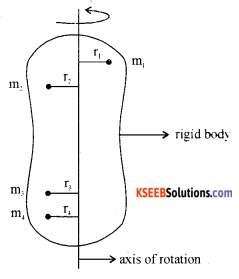Where , m1, m2, m3. … mn are mass of particles at perpendicular distances r1, r2, r3,……….rn respectively, from the axis of rotation.
∴Rotational K.E. of the body = Sum of linear K.E of all the particles of the rotating system.Question 23.
State Kepler’s laws of planetary motion.
Kepler’s I law (Law of orbit): All planets revolve in elliptical orbits with Sun as one of its foci.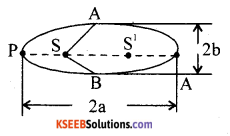2a – Major axis length
2b – Minor axis length
S-Sun at one focus P
S1 – The other focus of the ellipse
P – Perihelion position of the planet.
A – Apehelion position of the planet.

Kepler’s II law (Law of areas) : The line joining the planet and the Sun sweeps out equal areas in equal intervals of time.
$$\frac{\Delta \overrightarrow{\mathrm{A}}}{\Delta \mathrm{t}}=\frac{1}{2 \mathrm{m}} \overrightarrow{\mathrm{L}}$$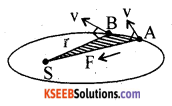Kepler’s III law (Law of periods) : The square of the period of revolution of a planet around the sun is directly proportional to the cube of the semi major axis of the ellipse.
i.e., T2 ∝ a3 so that for two planets
$$\left(\frac{T_{1}}{T_{2}}\right)^{2}=\left(\frac{a_{1}}{a_{2}}\right)^{3}$$

Question 24.
Calculate $$\frac{C_{p}}{C_{v}}$$ for monatomic gas.
$$\frac{C_{p}}{C_{v}}$$ = γ = 1 + $$\frac{2}{f}$$
where f= 3 for monatomic gas
Hence γ = 1+ $$\frac{2}{f}$$ = 1 + 0.667
i.e. γ = 1.667.
Hence $$\frac{C_{p}}{C_{v}}$$ for monatomic gas = 1.667Question 25.
Write stress-strain curve for a metal. What is proportional limit and yield point?
Statement: The ratio of stress to strain is a constant for a material within the elastic limit.Modulus of elasticity = $$\frac{\text { Stress }}{\text { Strain }}$$

Within the elastic limit, stress v/s strain is a straight line ‘A’ is the elastic limit upto which Hooke’s law is applicable. Beyond ‘B’ the yielding point, the wire extends but does not return to the initial state when the deforming force is removed. ‘F’ is the breaking point. ‘EF’ allows the material to be malleable and ‘DE’, ductile.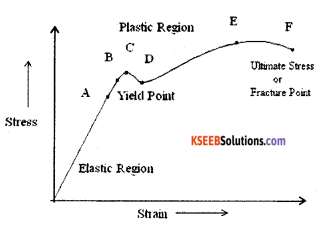Question 26.
State Bernoulli’s and write the equation principle.
Along a steamline, in a steady flow of non viscous fluid, potential energy, kinetic energy and pressure energy remain constant.
i.e., mgh + 1/2 mv2 + PV = K
i.e., mgh + 1/2 mv2 + Pm/ρ = K ÷ m
we get, gh + $$\frac{v^{2}}{2}+\frac{P}{\rho}$$ =K ÷ g
i.e., h + $$\frac{v^{2}}{2 g}+\frac{\mathrm{P}}{\rho g}$$ = K
where ‘h’ is called gravitational head, $$\frac{v^{2}}{2 g}$$ is velocity head and $$\frac{P}{\rho g}$$ is pressure head.
For horizontal Flow, $$\frac{\rho v^{2}}{2}$$ + P = K.
As the velocity of the fluid increases, pressure of the fluid decreases.

Part – D

IV. Answer any Two of the following questions: ( 2 × 5 = 10 )

Question 27.
Derive the equation x = v0t +$$\frac{1}{2}$$at2 using V-T graph.
A graph of velocity of a particle plotted along the Y-axis and time along the X-axis is known as v-t graph and the curve is known as velocity time curve.
Let ‘v0’ be the initial velocity of a particle. Let ‘a’ be the B uniform acceleration v – t graph gives a straight line with a constant slope, tanθ = m
i.e. a = $$\frac{v-v_{0}}{t}$$……..(1)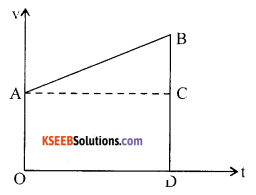From the figure OABD is a trapezium.
Area of trapezium OABD = Area of rectangle OACD + Area of triangle ACB
The second term on the right indicates the additional distance covered by the particle due to acceleration.
i.e., area of trapezium = (OA)(OD) + 1/2 (AC)(BC) = v0t + 1/2 t(v – v0) by using (1)
Area of trapezium = v0t + 1/2 at2
From the dimensional analysis. the right hand terms indicate the distance travelled. From the principle of homogeneity, the left hand side term should indicate the distance covered.
Hence area of the trapezium = x = v0t + 1/2 at2
For a uniform motion, a = 0 and x = v0t
For a particle starting from rest v0 = 0, x = 1/2 at2
in a vector form, $$\vec{x}=\vec{v}_{0} t+\frac{1}{2} \overrightarrow{a t}^{2}$$Question 28.
State and explain law of conservation of momentum with proof.
Statement: In an isolated system of collision of bodies, the total linear momentum before impact is equal to the total linear momentum after impact.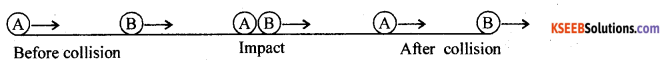Let m1 and m2 be the masses of two bodies moving along $$\vec{v}_{1 i}$$ and $$\vec{v}_{2 i}$$. Let $$\vec{v}_{1 f}$$ and be the $$\vec{v}_{2 f}$$ be final velocities after the impact.
At the time of impact the force of action acts on the body B and the force of reaction acts on A.
Applying Newton’s III law of motion
$$|Force of action on \mathrm{B}|=-| Force of reaction on \mathrm{A} |$$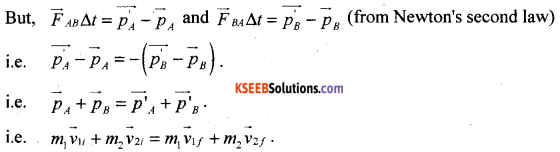This shows that the total final linear momentum of the isolated system equals its total initial momentum.

Question 29.
State and explain parallel axes theorem and perpendicular axes theorem.
Statement: The moment of inertia of a body about any axis is equal to the sum of the moment of inertia of the body about a parallel axis passing through the centre of mass and the product of mass of the body and square of the distance between the two parallel axes.
I = I0 + Md2.
Let ‘d’ be the distance between the two parallel axes. Let I be the moment of inertia about an axis passing through the centre of mass of a thin rod of length L.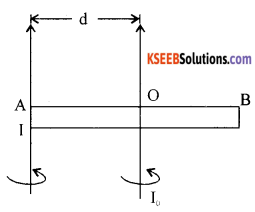We know that I0 = $$\frac{\mathrm{ML}^{2}}{12}$$ and d = $$\frac{\mathrm{L}}{2}$$
Hence moment of inertia at one end = $$\frac{M L^{2}}{12}+M\left(\frac{L}{2}\right)^{2}$$
I = $$\frac{M L^{2}}{4}\left[\frac{1}{3}+1\right]=\frac{M L^{2}}{3}$$

Statement : The moment of inertia about an axis perpendicular to two other axes acting in the same plane with their point of intersection being a point on it and the (third) axis passing through the common point, is equal to the sum of moments of inertia about the two axes.
e.g: Iz = Ix + Iy.
Let M be the mass of the disk of radius R.
M.I. about a point passing through the centre and perpendicular to the plane containing X and Y is I = $$\frac{M R^{2}}{2}$$
Since X and Y are in the same plane, Ix = Iy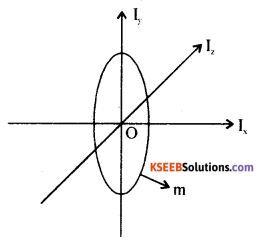∴ Iz = Ix + Iy becomes Iz = 2Ix
hence Ix = $$\frac{I_{Z}}{2}=\frac{M R^{2}}{4}$$
i.e. moment of inertia of a circular disc about the diameter $$\frac{I_{Z}}{2}=\frac{M R^{2}}{4}$$

V. Answer any Two of the following questions : ( 2 × 5 =10 )

Question 30.
Explain working of Carnot’s heat engine.
Working of Carnot’s engine:
Step 1 : The working substance (ideal gas) is enclosed ¡n a non-conducting wall and conducting bottom of a cylinder fitted with air tight non conducting piston. This is placed on the source having an infinite thermal capacity at a steady temperature.
The top surface is conducting and the rest non conducting. As a result, the gas expands isothermally. The work done
by the system.
Q = W1 = μRT1 log$$\frac{V_{2}}{V_{1}}$$(curve A B)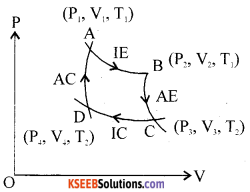Step 2 : The working substance is now placed on a non L conducting platform. as a result ofwhich no heat exchange takes place between the system and the surroundings. [he system expands adiabatically at the expense of its internal energy. The gas cools. The work done by the system.
$$\mathrm{W}_{2}=\frac{\mu \mathrm{R}}{\gamma-1}\left(\mathrm{T}_{1}-\mathrm{T}_{2}\right)$$(curve B C)

Step 3 : The working substance is now placed on the sink maintained at a steady low temperature T,K. The system undergoes isothermal compression at this temperature. The pressure of gas increases and volume decreases without any change in the internal energy and specific heat of gas remains at infinity.
$$\mathrm{W}_{3}=\mu \mathrm{RT}_{2} \log \left(\frac{\mathrm{V}_{4}}{\mathrm{V}_{3}}\right)$$ (curve C D)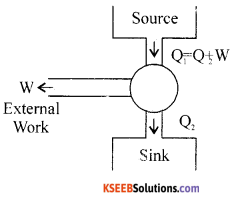Step 4: The working substance is placed on a non-conducting platform, Under thermal isolation. the system undergoes change in its internal energy and its specific heat remains at zero. Adiabatic compression results in increase in the pressure and temperature at the expense of work being done on the system. The system is allowed to reach its initial slate. This completes one cycle of operation. The area hounded by the curves gives the amount of heat converted into work.
$$\mathrm{W}_{4}=\frac{-\mu \mathrm{R}}{\gamma-1}\left(\mathrm{T}_{1}-\mathrm{T}_{2}\right)$$ (curve D A)
i.e., Net work done = W =W1 + W2 +W3 + W4
Work done = μR(T – T2) $$\log _{e}\left(\frac{V_{2}}{V_{1}}\right)$$
∴Efficiency η = $$\frac{\mathrm{w}}{\mathrm{Q}}=1-\frac{\mathrm{T}_{2}}{\mathrm{T}_{1}}$$Question 31.
Derive an expression for energy of a body which is in S.H.M.
We know that total energy of a particle executing SHM,
TE = KE + PE
where K.E= $$\frac{1}{2}$$ mv2
K.E = $$\frac{1}{2}$$mA2 cos2 (ωt + Φ)ω2
and P.E = $$\frac{1}{2}$$ ky2
i.e., P.E = $$\frac{1}{2}$$ mω2. A2sin2(ωt + Φ)
Hence T.E = $$\frac{1}{2}$$ mω2. A2 (cos2(cost + Φ) + sin2 (ωt + Φ))
or T.E = $$\frac{1}{2}$$ mω2. A2
Representing mω2 = k
we get TE = $$\frac{1}{2}$$ kA2
K.E of the particle=$$\frac{1}{2}$$ mω2. A2 – $$\frac{1}{2}$$ mω2. x2
= $$\frac{1}{2}$$ mω2(A2 -x2 )
where x = A cos (ωt + Φ)
Also K.E = $$\frac{1}{2}$$ mω2(A2)(1 – cos2(ωt + Φ))
i.e., K.E = $$\frac{1}{2}$$ mω2 A2sin2(ωt + Φ)
K(t) – K.E of a particle.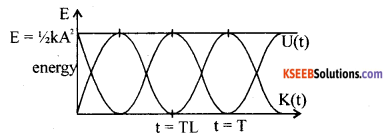U(t) of a particle.
T.E = K(t) + U(t) = $$\frac{1}{2}$$ kA2
where A – amplitude, k = mω2, m – mass of the particle
ω = angular frequency = $$\frac{2 \pi}{\mathrm{T}}$$
T = Period of SHM
TE = KE + PE = $$\frac{1}{2}$$mω2 A2 cos2 (ωt + Φ) + $$\frac{1}{2}$$ mω2 A2sin2(ωt + Φ) = $$\frac{1}{2}$$ mω2 A2

Question 32.
What is Doppler effect of sound? Derive expression for apparent frequency of sound when source is moving away from stationary listener.
The phenomenon of apparent change in frequency due to relative motion between the source of sound and the listener (observer) is known as Doppler effect.
Let S1 be the position of the source at t = 0. Let L be the distance b/w the observed and the source at S,
Let v be the velocity of sound in air.
Time taken for sound (say crest) to reach the observer
t1 = $$\frac{L}{v}$$ ……..(1)
Let the source travel further from S1, and occupy the position S, away from a stationary observer.
distance S1S2 = vsT0
For a crest at S1 to reach the observer, it takes the time t2 = T0 + $$\frac{\mathrm{L}+\mathrm{v}_{\mathrm{s}} \mathrm{T}_{0}}{\mathrm{v}}$$ ………(2)
At time nT, the source emits its (n +1)th crest and this reaches the observer at time.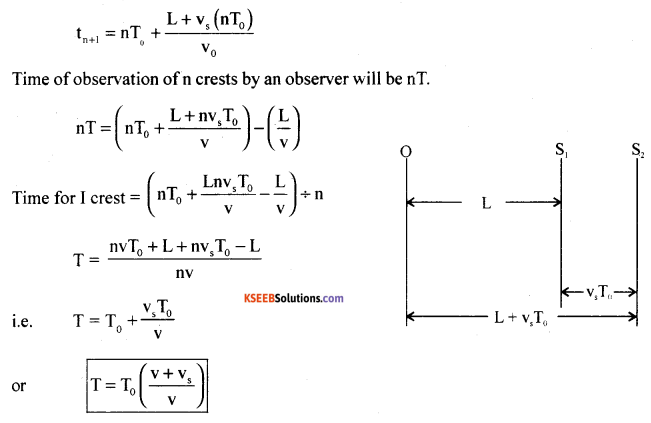Apparent frequency of sound heard by a stationary observer for a source of sound moving away from him
f = f0 ($$\frac{\mathbf{v}}{\mathbf{v}+\mathbf{v}_{\mathbf{s}}}$$)
and apparent wavelength X = $$\frac{v+v_{s}}{f_{0}}$$, change (increase) in wavelength.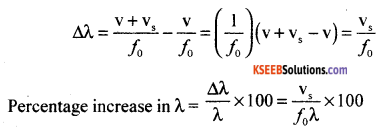For a source moving towards the observer,change vs to – vs
Then f = f0 ($$\frac{\mathbf{v}}{\mathbf{v}-\mathbf{v}_{\mathrm{s}}}$$)
Apparent wave length = $$\frac{v-v_{S}}{f_{0}}$$, here λ<λ0

VI. Answer any Three of the following questions : ( 3 × 5 = 15 )

Question 33.
A body is projected at an angle of 30° with the horizontal and with a velocity of 39.2 ms-1. Find,
(a) Time of flight (T)
(b) Range (R)
(c) Maximum height (H)
Given θ = 30°, u = 39.2 ms-1.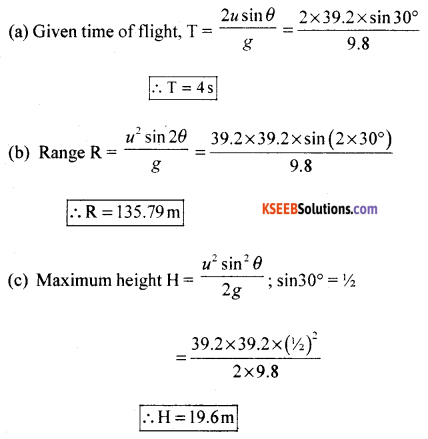Question 34.
A body of mass 5 kg moving with a velocity of 6 ms-1 collide with another body of mass 2 kg which is at rest. Afterwards they move in the same direction as before. If the velocity of the body of mass 2 kg is 10 ms-1, find the velocity and kinetic energy of the body of mass 5 kg.
Given m1 = 5 kg, u, = 6 ms-1.
m2 = 2 kg, u2 = 0
v2 = 10 ms-1, v1 = ?, KE1 =?.
Applying m1u1 + m2u2 = m1v1 + m2v2 we get
5 × 6 + 2 × 0 = 5v1 + 2 × 10
i.e., 5v1 = 30 – 20 = 10
or v1 = $$\frac{10}{5}$$ = 2ms-1 in the direction of $$\vec{v}_{2}$$
Kinetic energy of m1 = $$\frac{1}{2} m_{1} v_{1}^{2}=\frac{1}{2} \times 5 \times 2 \times 2$$
K.E = 10J

Question 35.
Find the potential energy of a system of four particles each of mass 5 kg placed at the vertices of a square of side 2m.
PE of the system = $$\frac{-4 G m^{2}}{a}-\frac{2 G m^{2}}{\sqrt{2} a}$$
where $$\sqrt{2} a$$ =diagonal of the square.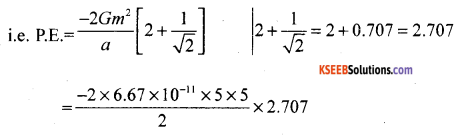= -451.39 × 1011J
PE = -4.514 × 10-9J

Question 36.
Two identical bars each of length l = 0.1 m and area A = 0.02 m2, one is iron of thermal conductivity K1 = 79 Wm-1 K-1 and another of brass of thermal conductivity K2 = 10 Wm-1 K-1 are soldered end to end. Free end of iron bar is at 373K and of brass bar is at 273K. Find the temperature of the junction.
Given A1 = A2 = A, l1 = l2 = l; K= 79 Wm-1 K-1.
K = 109 Wm-1K-1, θ1 = 373 K,  θ2 = 273K , θ = ?
Since θ1 = θ2
$$\frac{K_{1} A\left(\theta_{1}-\theta_{2}\right) t}{l_{1}}=\frac{K_{2} A\left(\theta-\theta_{2}\right) t}{l_{2}}$$
∴K11 – θ) = K2 (θ – θ2)
→ 79(373 – θ)= 109(θ – 273)
→ 29467 – 79θ = -109θ – 29757
i.e., 188θ = 59224 →
θ = $$\frac{59224}{188}$$ = 315.02
∴temperature of the junction is 315.02 K.Question 37.
A wave travelling in a string is described, by an equation y(x, t) = 0.005 sin(80πt – 3πx) all are in SI unit. Find (a) amplitude (A) (b) Wave length (A) (c) Period (T).
Given y(x, t) = 0.005 sin (80πt – 3πx); A =,?, λ = ?, T = ?, f = ?
comparing the given equation y (x, t) A sin (ωt – kx) with the equation
(i)A 0.005m or A = 5 x 10-3m.
(ii) k = 3 rad m-1.
i.e., k = 3 x 3.142 = 12.726 rad m-1
but k = $$\frac{2 \pi}{\lambda}$$
3 = $$\frac{2 \pi}{\lambda}$$
or λ = ($$\frac{2}{3}$$) = 0.667 m
(iii) ω = 80π
i.e., 2πf = 80π
∴ f = 40 Hz
(iv) T= $$\frac{1}{f}$$
∴T= $$\frac{1}{40}$$ =0.025 s
or T=25 × 10-3s
or T = 25 ms